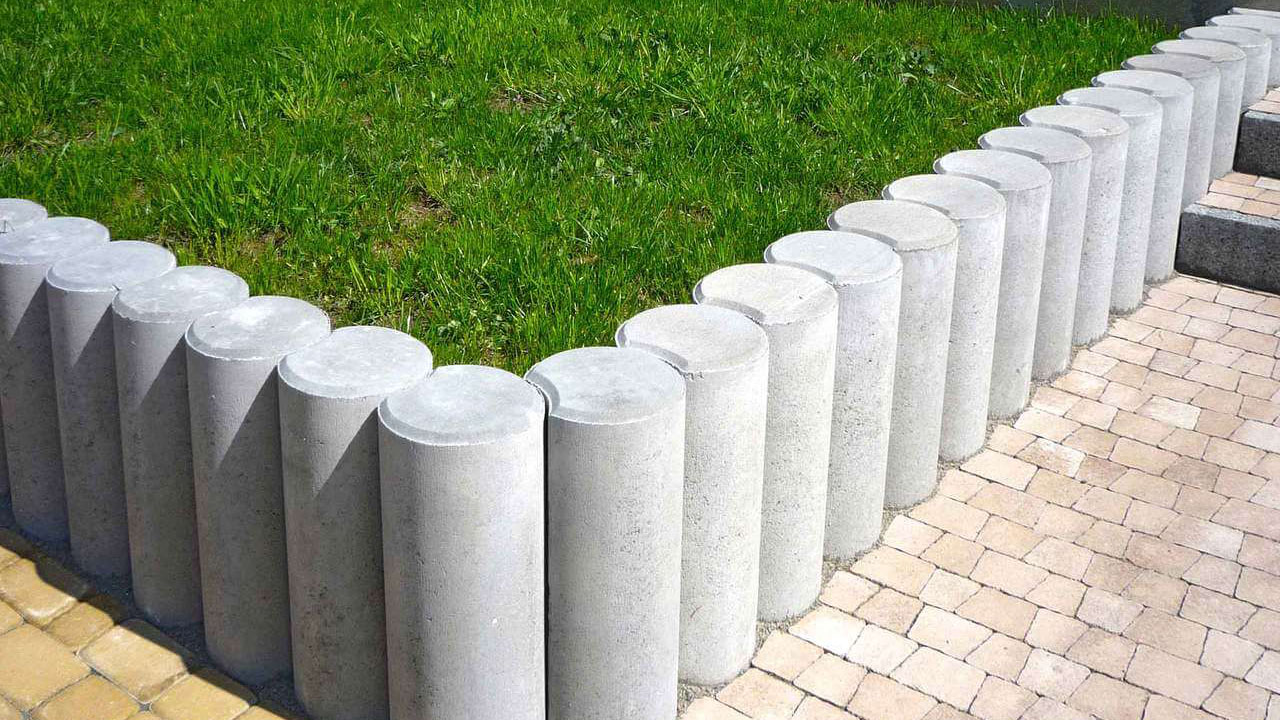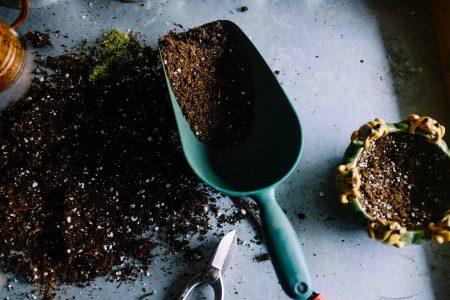Garden borders of wood, rock, brick or plastic look great on garden landscape and help highlight its prominent features and enhance its natural beauty. Garden borders can be of different shapes and sizes depending on the area of land around the house. Furthermore, they are a practical way to reduce and control weed and grass growing in the flowerbed. Most landscapers use borders to tie different elements of a garden together with the materials used around the property. So making calculations for a garden border involves using simple tools and a little math.

A conversion chart helps for square footage calculations. This is why we have provided charts for converting between different units of measurement. Whether it is the area of a rectangular border, square border or the area of a circular border you can utilize our calculators and figure out how much border material you need.1. Area of a Rectangular Border
The area of a rectangle is the space contained within a set of lines. To find out the area of a rectangle border, we need to subtract the area of the inner rectangle from the area of the outer rectangle. These lines should be measured in feet (ft) for square footage calculations and if needed, converted to inches (in), yards (yd), centimetres (cm), millimetres (mm) and metres (m).

#### Formula:

Inner area = (Length x Width)
Total area = (Length + (2 x Width of border)) x (Width + (2 x Width of border))
Area of a Rectangle border = Total area – Inner area
Answer = (Side length x Side length) square area
Abbreviations of unit area: ft2, in2, yd2, cm2, mm2, m2

2. Area of a Circular Border
The area of a circle is the space contained within its circumference (outer perimeter). To find out the area of a circle border, we need to know its inner diameter and the width of the circular border. The diameter and border width should be measured in feet (ft) for square footage calculations and if needed, converted to inches (in), yards (yd), centimetres (cm), millimetres (mm) and metres (m).

#### Formula:

Outer diameter = Inner diameter + (2 x Border width)
Outer area = π x (Outer diameter/2)^2
Inner area = π x (Inner diameter/2)^2
Area of a Circle Border = Outer area – Inner area
π = 3.142
Answer = (Outer area – Inner area) square area
Abbreviations of unit area: ft2, in2, yd2, cm2, mm2, m2Steps to make the measurement:

• Start with measuring the entire outside edge of your garden. You can make a rough drawing of its shape on a piece of paper and mark your readings on this drawing. Writing down the readings is important. Otherwise you risk miscalculating the border area as you might not remember the readings incorrectly.
• Place a length of twine around the edge of an irregularly shaped garden. Make sure to include all curves and turns. You can also use this method for a square or rectangular garden for a more accurate measurement. Then measure this twine with a measuring tape. This is the total length of the outside of your garden border.
• Next you have to measure the length of one piece of garden border material. This can be any kind of material or a combination of materials: individual bricks, plastic edging, railroad ties or whatever material you plan to use. Note down these measurements.
• Remember to add 2 to 4 feet to this measurement. This extra material will make up for mistakes in measurement, errors in cutting, broken corners, curves which might fit different materials at different lengths that require adjustments during installation.
• The last step in calculation is to divide the total length of the garden border by the measurement of the individual border pieces. This figure provides the total number of sections, bricks or pieces of material required. You can calculate the area of the rectangular border in square feet, in square inches, square yards, square centimetres, square millimetres and square meters using our online conversion tables.

So keep building and leave the calculation part to us! Want more calculators? Let us know which calculators you need and we will try our best to add them to our website. Write to us.